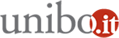# Statistics seminar: “Inequalities: Theory of majorization and its applications”

Seminario di Statistica

20/05/2013 dalle 14:30 alle 16:30

Dove Dipartimento di Scienze Statistiche - Via Belle Arti 41 - Aula IAggiungi l'evento al calendario

Relatore

Ingram Olkin

Department of Statistics, Stanford University

Abstract

There are many theories of “equations”: linear equations, differential equations, functional equations, and more. However, there is no central theory of “inequations.” There are several general themes that lead to many inequalities. One such theme is convexity. Another theme is majorization, which is a particular partial order. What is important in this context is that the partial order have lots of examples, and that the order-preserving functions be a rich class. In this case majorization arises in many fields --- in mathematics: geometry, numerical analysis, graph theory; in other fields: physics, chemistry, political science, economics. In this talk we describe the origins of majorization and many examples of majorization and its consequences.